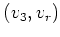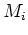Previous: 2.2.1.2 Physical Quantities Up: 2.2 The Method of Next: 2.2.2.1 Even Moments

## 2.2.2 Hierarchy of Moment Equations

We get the equation system corresponding to a set of observables by multiplying the Boltzmann equation with the observable as a weight function and integrating over the cylinder-symmetric phase space. Due to the structure of the Boltzmann equation additional moments not stemming from the set of observables appear in the equations.

In the parabolic band case we use the set of weight functions. For the unknowns we use the corresponding moments. This is done in the calculations below.

SubsectionsPrevious: 2.2.1.2 Physical Quantities Up: 2.2 The Method of Next: 2.2.2.1 Even Moments